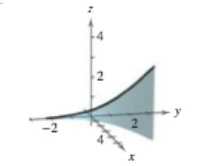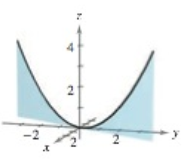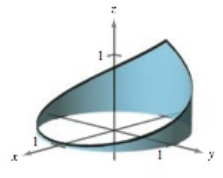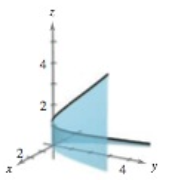Chapter 12.1, Problem 19E

Chapter
Section
Textbook Problem

# MatchingIn Exercises 19–22, match the equation with its graph. [The graphs are labeled (a), (b), (c), and (d).](a)(b)(c)(d)r ( t ) = t i + 2 t j + t 2 k ,       − 2 ≤ t ≤ 2

To determine
Match the graph of the function, r(t)=t i+2t j+t2 k from the provided four options.

Explanation

Given:

The equation of vector value function, r(t)=t i+2t j+t2 k, where 2t2. The graph are given below,

Explanation:

Consider the provided vector value function equation,

r(t)=t i+2t j+t2 k where 2t2

Make the space curve of the provided vector equation by the use of maple software:

Step1: First write the library function with(VectorCalculus):.

Step2: Define the position vector in Cartesian coordinate system,

S1:=PositionVecor([t,2t,t2],cartesian)

Step3: Press Enter button

### Still sussing out bartleby?

Check out a sample textbook solution.

See a sample solution

#### The Solution to Your Study Problems

Bartleby provides explanations to thousands of textbook problems written by our experts, many with advanced degrees!

Get Started

#### Find more solutions based on key concepts